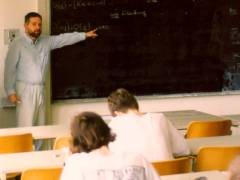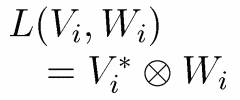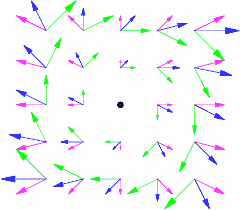# Hans Havlicek: Multilinear Algebra

This is a series of lectures and exercises for graduate students of mathematics. We present an introduction to the basic concepts of multilinear algebra.

## Contents

The subsequent list refers to my lectures in summer 2000 (chapters 1-3) and winter 2001 (chapters 4 and 5).

The first part is due to be read in summer 2008.A lecture on multilinear algebra in June 1998Rotation of a vector field

## 1. Tensor Products

• Multilinear mappings of vector spaces
• Existence and universal property of the tensor product
• Commutativity and associativity of the tensor product
• The tensor product in terms of coordinates
• Tensor products and spaces of linear mappings
• Kronecker product of linear mappings (matrices)
• Contraction
• Lowering and raising of indices
• Duality

## 2. Tensor Algebras

• Covariant, contravariant and mixed tensors
• Classical definition and notation of a tensor in terms of coordinates
• Structure tensor of an algebra
• Mixed tensor algebra
• Universal property of the tensor algebra

## 3. Exterior Algebras

• Exterior powers and p-vectors
• Grassmann coordinates of subspaces
• Alternation operator
• Exterior powers of linear mappings
• Exterior algebra
• Duality and p-forms
• Exterior algebra
• Decomposable p-vectors

## 4. Clifford Algebras

• Clifford mappings
• Clifford mappings and exterior algebra
• Clifford Algebras
• Dimension of a Clifford algebra
• Examples of Clifford algebras
• Structures on a Clifford algebra

## 5. Spinors

• Clifford groups and spin groups
• Isometries
• The centre of a Clifford algebra
• Semisimple modules and representation of algebras
• Spaces of spinors